# ENTHALPY CHANGES A guide for A level students

• Slides: 37ENTHALPY CHANGES A guide for A level students KNOCKHARDY PUBLISHINGENTHALPY CHANGES INTRODUCTION This Powerpoint show is one of several produced to help students understand selected topics at AS and A 2 level Chemistry. It is based on the requirements of the AQA and OCR specifications but is suitable for other examination boards. Individual students may use the material at home for revision purposes or it may be used for classroom teaching if an interactive white board is available. Accompanying notes on this, and the full range of AS and A 2 topics, are available from the KNOCKHARDY SCIENCE WEBSITE at. . . www. argonet. co. uk/users/hoptonj/sci. htm Navigation is achieved by. . . either clicking on the grey arrows at the foot of each page or using the left and right arrow keys on the keyboardENTHALPY CHANGES CONTENTS • Thermodynamics • Enthalpy changes • Standard enthalpy values • Standard enthalpy of formation • Standard enthalpy of combustion • Enthalpy of neutralisation • Bond dissociation enthalpy • Hess’s law • Calculating enthalpy changes using bond enthalpies • Calculating enthalpy changes using enthalpy of formation values • Calculating enthalpy changes using enthalpy of combustion values • Practical measurement • Check listENTHALPY CHANGES Before you start it would be helpful to… • be able to balance equations • be confident with simple arithmetical operations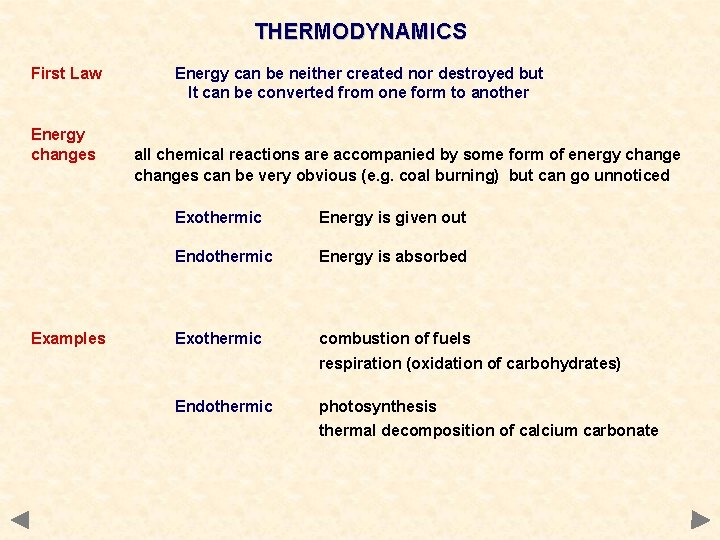THERMODYNAMICS First Law Energy changes Examples Energy can be neither created nor destroyed but It can be converted from one form to another all chemical reactions are accompanied by some form of energy changes can be very obvious (e. g. coal burning) but can go unnoticed Exothermic Energy is given out Endothermic Energy is absorbed Exothermic combustion of fuels respiration (oxidation of carbohydrates) Endothermic photosynthesis thermal decomposition of calcium carbonate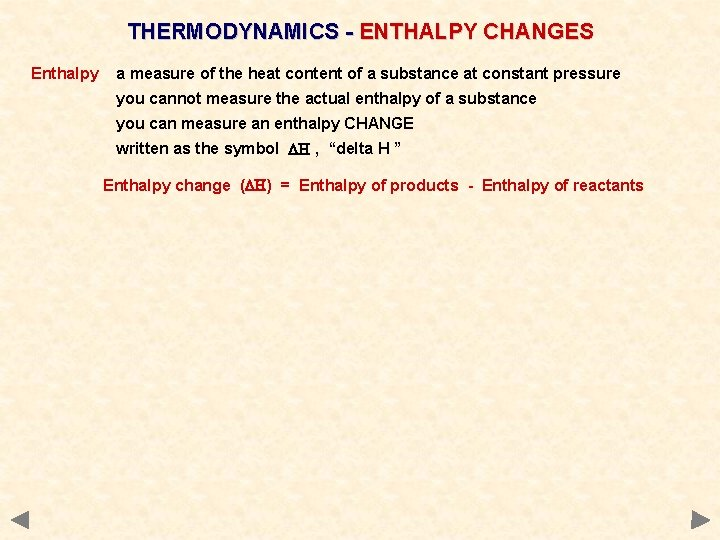THERMODYNAMICS - ENTHALPY CHANGES Enthalpy a measure of the heat content of a substance at constant pressure you cannot measure the actual enthalpy of a substance you can measure an enthalpy CHANGE written as the symbol DH , “delta H ” Enthalpy change (DH) = Enthalpy of products - Enthalpy of reactants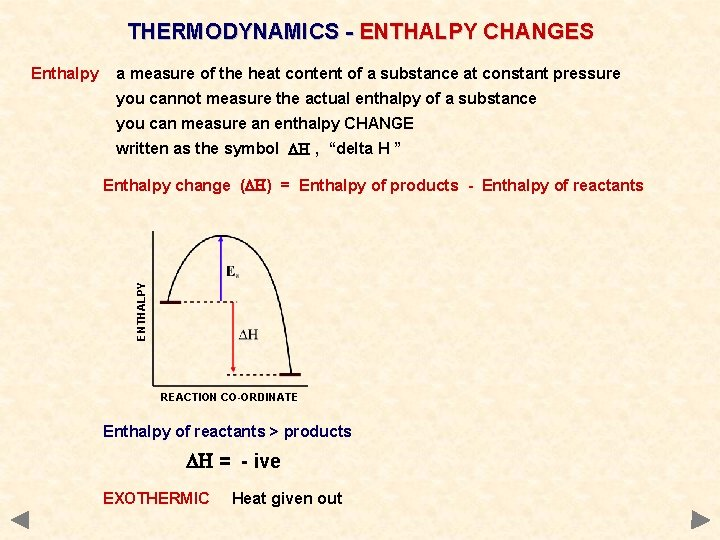THERMODYNAMICS - ENTHALPY CHANGES a measure of the heat content of a substance at constant pressure you cannot measure the actual enthalpy of a substance you can measure an enthalpy CHANGE written as the symbol DH , “delta H ” Enthalpy change (DH) = Enthalpy of products - Enthalpy of reactants ENTHALPY Enthalpy REACTION CO-ORDINATE Enthalpy of reactants > products DH = - ive EXOTHERMIC Heat given out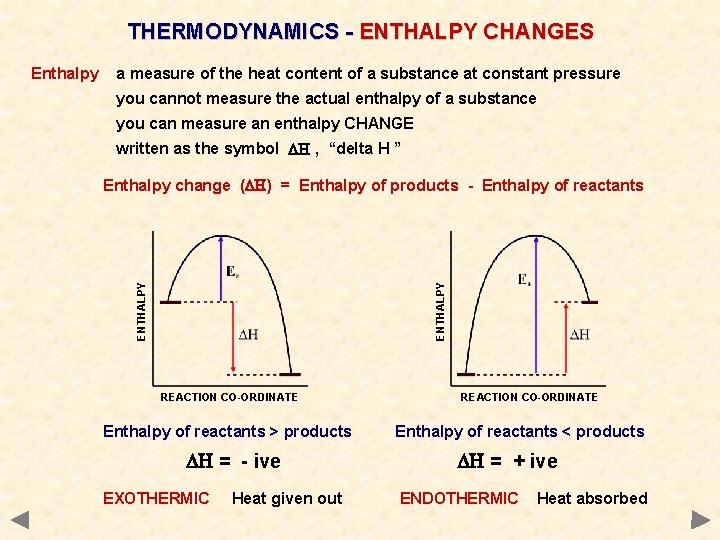THERMODYNAMICS - ENTHALPY CHANGES a measure of the heat content of a substance at constant pressure you cannot measure the actual enthalpy of a substance you can measure an enthalpy CHANGE written as the symbol DH , “delta H ” ENTHALPY Enthalpy change (DH) = Enthalpy of products - Enthalpy of reactants ENTHALPY Enthalpy REACTION CO-ORDINATE Enthalpy of reactants > products DH = - ive EXOTHERMIC Heat given out REACTION CO-ORDINATE Enthalpy of reactants < products DH = + ive ENDOTHERMIC Heat absorbedSTANDARD ENTHALPY CHANGES Why a standard? enthalpy values vary according to the conditions a substance under these conditions is said to be in its standard state. . . Pressure: A stated temperature 100 k. Pa (1 atmosphere) usually 298 K (25°C) • as a guide, just think of how a substance would be under normal lab conditions • assign the correct subscript [(g), (l) or (s) ] to indicate which state it is in • any solutions are of concentration 1 mol dm-3 • to tell if standard conditions are used we modify the symbol for DH. Enthalpy Change Standard Enthalpy Change (at 298 K)STANDARD ENTHALPY OF FORMATION Definition The enthalpy change when ONE MOLE of a compound is formed in its standard state from its elements in their standard states. Symbol DH°f Values Usually, but not exclusively, exothermic Example(s) C(graphite) + O 2(g) ———> CO 2(g) H 2(g) + ½O 2(g) ———> 2 C(graphite) + ½O 2(g) + Notes H 2 O(l) 3 H 2(g) ———> C 2 H 5 OH(l) Only ONE MOLE of product on the RHS of the equation Elements In their standard states have zero enthalpy of formation. Carbon is usually taken as the graphite allotrope.STANDARD ENTHALPY OF COMBUSTION Definition The enthalpy change when ONE MOLE of a substance undergoes complete combustion under standard conditions. All reactants and products are in their standard states. Symbol DH°c Values Always exothermic Example(s) C(graphite) + O 2(g) ———> CO 2(g) H 2(g) + ½O 2(g) ———> H 2 O(l) C 2 H 5 OH(l) + 3 O 2(g) ———> 2 CO 2(g) + 3 H 2 O(l) Notes Always only ONE MOLE of what you are burning on the LHS of the equation To aid balancing the equation, remember. . . you get one carbon dioxide molecule for every carbon atom in the original and one water molecule for every two hydrogen atoms When you have done this, go back and balance the oxygen.BOND DISSOCIATION ENTHALPY Definition Energy required to break ONE MOLE of gaseous bonds to form gaseous atoms. Values Endothermic - Energy must be put in to break any chemical bond Examples Cl 2(g) Notes • • ———> 2 Cl(g) O-H(g) ———> O(g) + H(g) strength of bonds also depends on environment; MEAN values quoted making bonds is exothermic as it is the opposite of breaking a bond for diatomic gases, bond enthalpy = 2 x enthalpy of atomisation smaller bond enthalpy = weaker bond = easier to break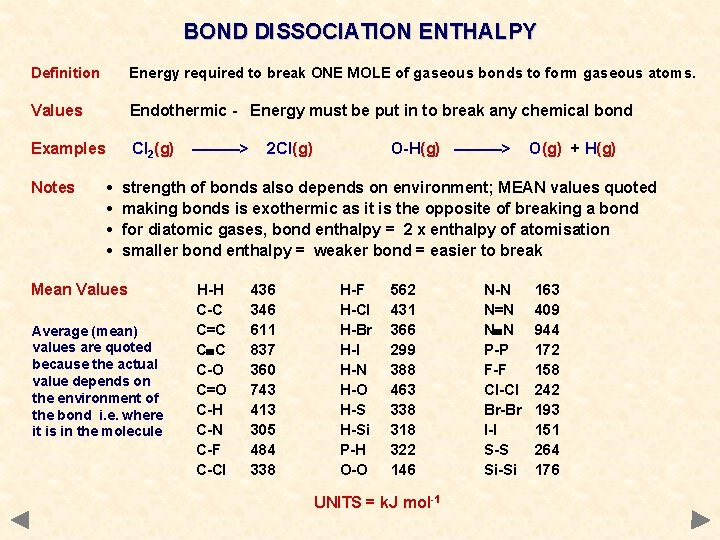BOND DISSOCIATION ENTHALPY Definition Energy required to break ONE MOLE of gaseous bonds to form gaseous atoms. Values Endothermic - Energy must be put in to break any chemical bond Examples Cl 2(g) Notes • • ———> 2 Cl(g) O-H(g) ———> O(g) + H(g) strength of bonds also depends on environment; MEAN values quoted making bonds is exothermic as it is the opposite of breaking a bond for diatomic gases, bond enthalpy = 2 x enthalpy of atomisation smaller bond enthalpy = weaker bond = easier to break Mean Values Average (mean) values are quoted because the actual value depends on the environment of the bond i. e. where it is in the molecule H-H C-C C=C C C C-O C=O C-H C-N C-F C-Cl 436 346 611 837 360 743 413 305 484 338 H-F H-Cl H-Br H-I H-N H-O H-Si P-H O-O 562 431 366 299 388 463 338 318 322 146 UNITS = k. J mol-1 N-N N=N N N P-P F-F Cl-Cl Br-Br I-I S-S Si-Si 163 409 944 172 158 242 193 151 264 176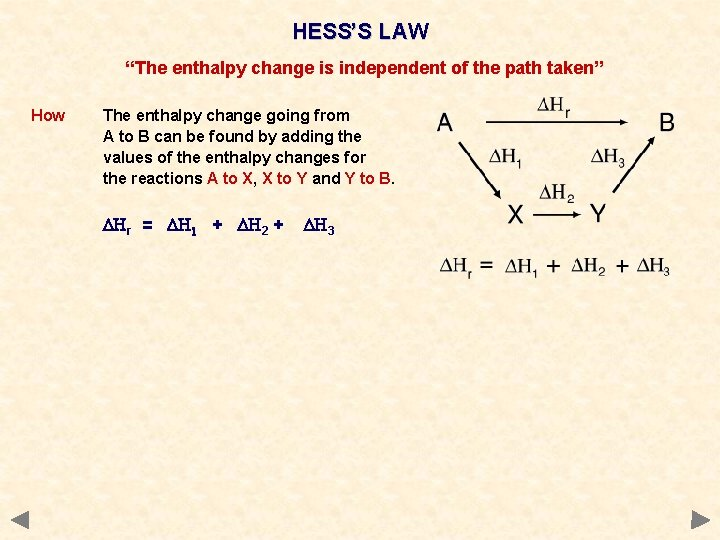HESS’S LAW “The enthalpy change is independent of the path taken” How The enthalpy change going from A to B can be found by adding the values of the enthalpy changes for the reactions A to X, X to Y and Y to B. DHr = DH 1 + DH 2 + DH 3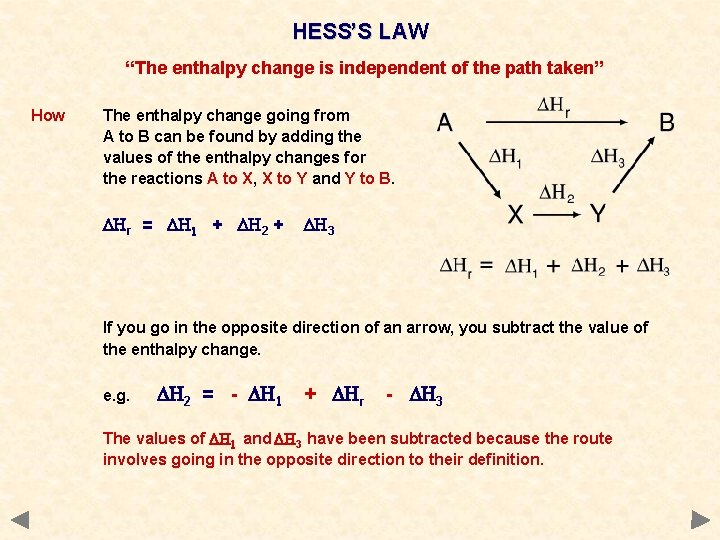HESS’S LAW “The enthalpy change is independent of the path taken” How The enthalpy change going from A to B can be found by adding the values of the enthalpy changes for the reactions A to X, X to Y and Y to B. DHr = DH 1 + DH 2 + DH 3 If you go in the opposite direction of an arrow, you subtract the value of the enthalpy change. e. g. DH 2 = - DH 1 + DHr - DH 3 The values of DH 1 and DH 3 have been subtracted because the route involves going in the opposite direction to their definition.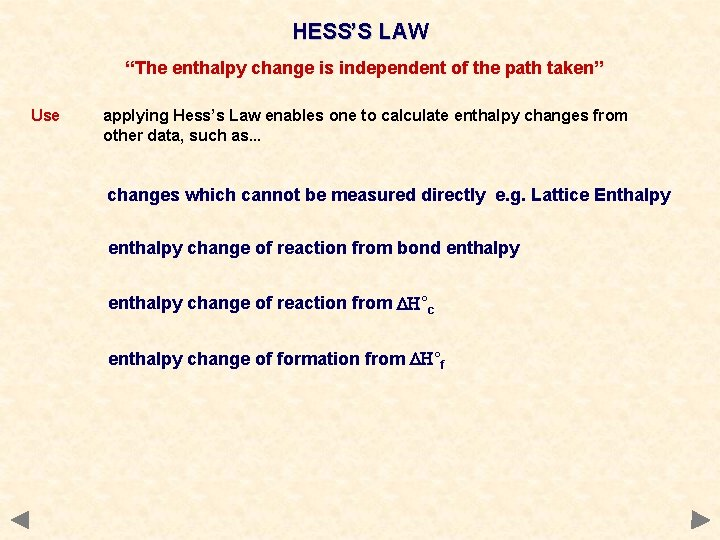HESS’S LAW “The enthalpy change is independent of the path taken” Use applying Hess’s Law enables one to calculate enthalpy changes from other data, such as. . . changes which cannot be measured directly e. g. Lattice Enthalpy enthalpy change of reaction from bond enthalpy change of reaction from DH°c enthalpy change of formation from DH°f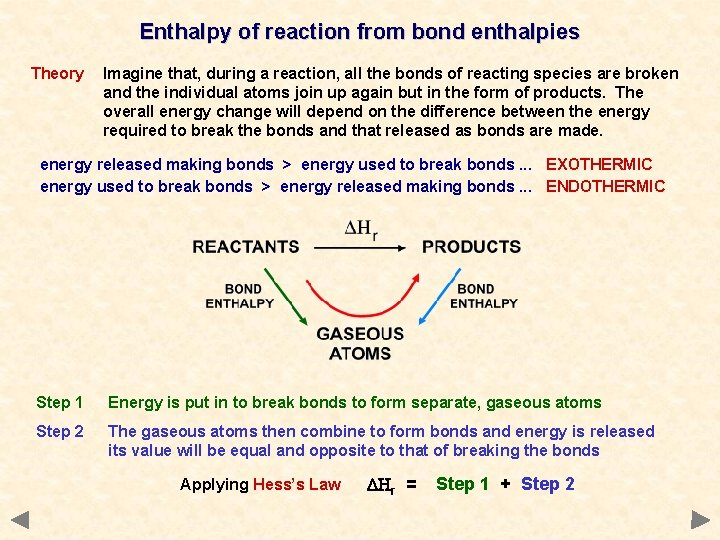Enthalpy of reaction from bond enthalpies Theory Imagine that, during a reaction, all the bonds of reacting species are broken and the individual atoms join up again but in the form of products. The overall energy change will depend on the difference between the energy required to break the bonds and that released as bonds are made. energy released making bonds > energy used to break bonds. . . EXOTHERMIC energy used to break bonds > energy released making bonds. . . ENDOTHERMIC Step 1 Energy is put in to break bonds to form separate, gaseous atoms Step 2 The gaseous atoms then combine to form bonds and energy is released its value will be equal and opposite to that of breaking the bonds Applying Hess’s Law DHr = Step 1 + Step 2Enthalpy of reaction from bond enthalpies Alternative view Step 1 Energy is put in to break bonds to form separate, gaseous atoms. Step 2 Gaseous atoms then combine to form bonds and energy is released; its value will be equal and opposite to that of breaking the bonds DHr = Step 1 - ATOMS SUM OFTHE BOND ENTHALPIES OF THE REACTANTS DH Step 2 Because, in Step 2 the route involves going in the OPPOSITE DIRECTION to the defined change of bond enthalpy, it’s value is subtracted. SUM OFTHE BOND ENTHALPIES OF THE PRODUCTS DH = bond enthalpies – bond enthalpies of reactants of products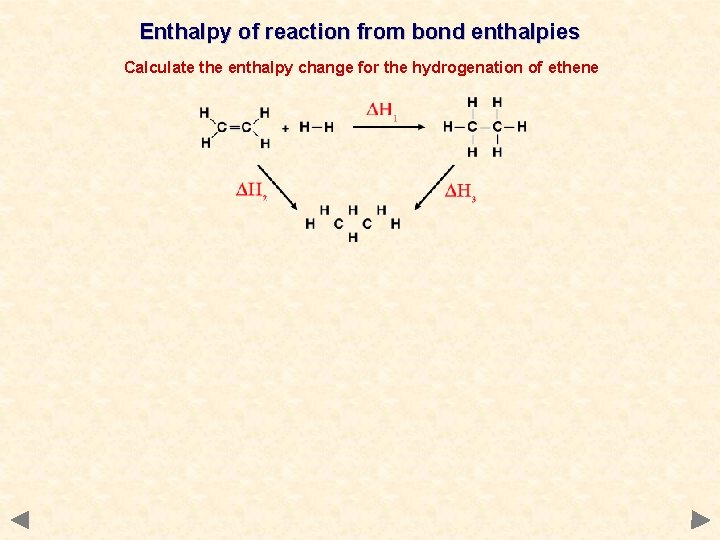Enthalpy of reaction from bond enthalpies Calculate the enthalpy change for the hydrogenation of ethene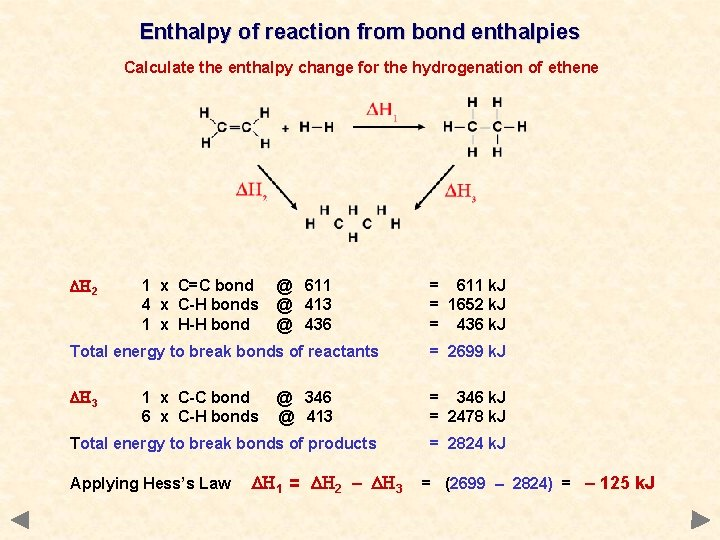Enthalpy of reaction from bond enthalpies Calculate the enthalpy change for the hydrogenation of ethene DH 2 1 x C=C bond 4 x C-H bonds 1 x H-H bond @ 611 @ 413 @ 436 = 611 k. J = 1652 k. J = 436 k. J Total energy to break bonds of reactants = 2699 k. J DH 3 = 346 k. J = 2478 k. J 1 x C-C bond 6 x C-H bonds @ 346 @ 413 Total energy to break bonds of products Applying Hess’s Law DH 1 = DH 2 – DH 3 = 2824 k. J = (2699 – 2824) = – 125 k. J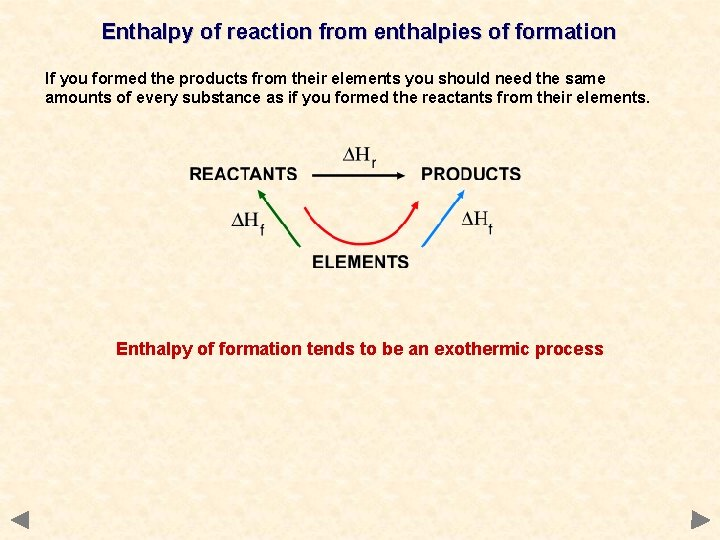Enthalpy of reaction from enthalpies of formation If you formed the products from their elements you should need the same amounts of every substance as if you formed the reactants from their elements. Enthalpy of formation tends to be an exothermic process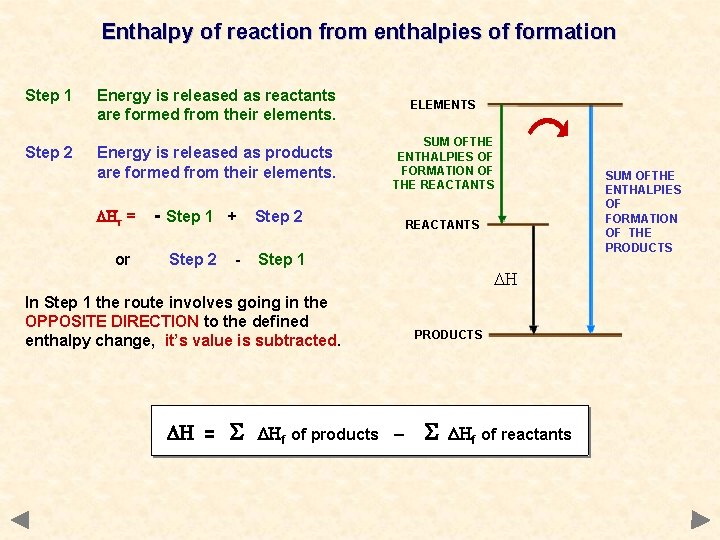Enthalpy of reaction from enthalpies of formation Step 1 Energy is released as reactants are formed from their elements. ELEMENTS Step 2 Energy is released as products are formed from their elements. SUM OFTHE ENTHALPIES OF FORMATION OF THE REACTANTS DHr = or - Step 1 + Step 2 - Step 1 In Step 1 the route involves going in the OPPOSITE DIRECTION to the defined enthalpy change, it’s value is subtracted. DH = DHf of products – REACTANTS DH PRODUCTS DHf of reactants SUM OFTHE ENTHALPIES OF FORMATION OF THE PRODUCTSEnthalpy of reaction from enthalpies of formation Sample calculation Calculate the standard enthalpy change for the following reaction, given that the standard enthalpies of formation of water, nitrogen dioxide and nitric acid are -286, +33 and -173 k. J mol-1 respectively; the value for oxygen is ZERO as it is an element 2 H 2 O(l) DH = + 4 NO 2(g) + O 2(g) DHf of products – ———> 4 HNO 3(l) DHf of reactants By applying Hess’s Law. . . The Standard Enthalpy of Reaction DH°r will be. . . PRODUCTS REACTANTS [ 4 x DHf of HNO 3 ] minus DH°r = 4 x (-173) - [ (2 x DHf of H 2 O) + (4 x DHf of NO 2) + (1 x DHf of O 2) ] 2 x (-286) ANSWER = - 252 k. J + 4 x (+33) + 0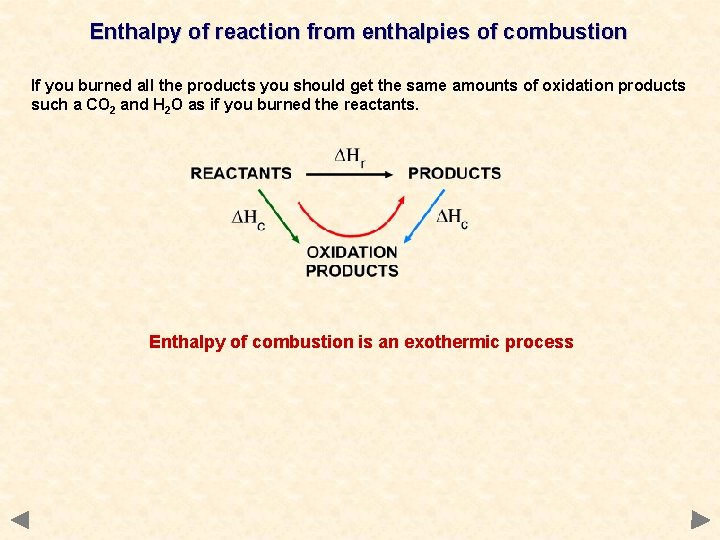Enthalpy of reaction from enthalpies of combustion If you burned all the products you should get the same amounts of oxidation products such a CO 2 and H 2 O as if you burned the reactants. Enthalpy of combustion is an exothermic processEnthalpy of reaction from enthalpies of combustion Step 1 Energy is released as reactants undergo combustion. Step 2 Energy is released as products undergo combustion. DHr = Step 1 - Step 2 Because, in Step 2 the route involves going in the OPPOSITE DIRECTION to the defined change of Enthalpy of Combustion, it’s value is subtracted. DH = DHc of reactants – REACTANTS DH PRODUCTS SUM OFTHE ENTHALPIES OF COMBUSTION OF THE PRODUCTS OXIDATION PRODUCTS DHc of products SUM OFTHE ENTHALPIES OF COMBUSTION OF THE REACTANTSEnthalpy of reaction from enthalpies of combustion Sample calculation Calculate the standard enthalpy of formation of methane; the standard enthalpies of combustion of carbon, hydrogen and methane are -394, -286 and -890 k. J mol-1. C(graphite) DH = + 2 H 2(g) DHc of reactants – ———> CH 4(g) DHc of products By applying Hess’s Law. . . The Standard Enthalpy of Reaction DH°r will be. . . REACTANTS PRODUCTS [ (1 x DHc of C) + (2 x DHc of H 2) ] DH°r = 1 x (-394) + 2 x (-286) minus [ 1 x DHc of CH 4 O ] - 1 x (-890) ANSWER = - 74 k. J mol-1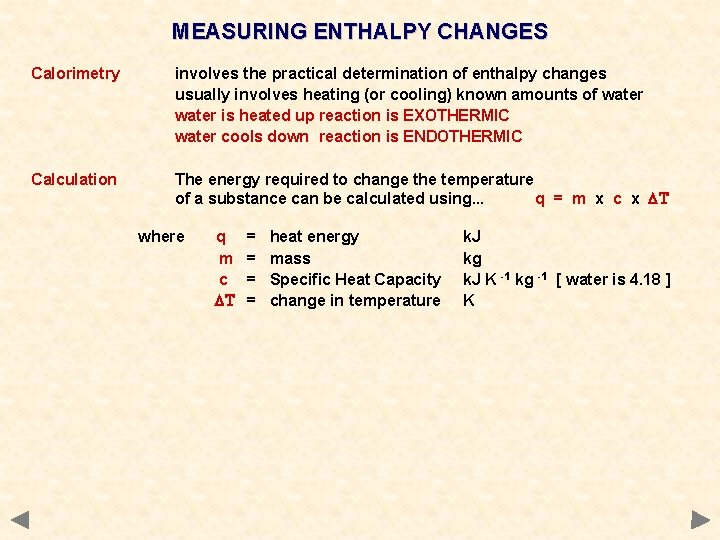MEASURING ENTHALPY CHANGES Calorimetry involves the practical determination of enthalpy changes usually involves heating (or cooling) known amounts of water is heated up reaction is EXOTHERMIC water cools down reaction is ENDOTHERMIC Calculation The energy required to change the temperature of a substance can be calculated using. . . q = m x c x DT where q m c DT = = heat energy mass Specific Heat Capacity change in temperature k. J kg k. J K -1 kg -1 [ water is 4. 18 ] K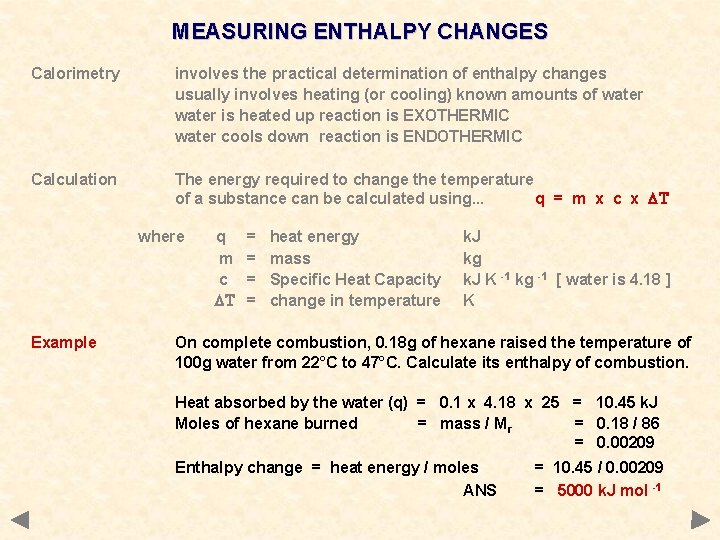MEASURING ENTHALPY CHANGES Calorimetry involves the practical determination of enthalpy changes usually involves heating (or cooling) known amounts of water is heated up reaction is EXOTHERMIC water cools down reaction is ENDOTHERMIC Calculation The energy required to change the temperature of a substance can be calculated using. . . q = m x c x DT where Example q m c DT = = heat energy mass Specific Heat Capacity change in temperature k. J kg k. J K -1 kg -1 [ water is 4. 18 ] K On complete combustion, 0. 18 g of hexane raised the temperature of 100 g water from 22°C to 47°C. Calculate its enthalpy of combustion. Heat absorbed by the water (q) = 0. 1 x 4. 18 x 25 = 10. 45 k. J Moles of hexane burned = mass / Mr = 0. 18 / 86 = 0. 00209 Enthalpy change = heat energy / moles ANS = 10. 45 / 0. 00209 = 5000 k. J mol -1MEASURING ENTHALPY CHANGES Example 1 - graphical The temperature is taken every half minute before mixing the reactants. Reactants are mixed after three minutes. Further readings are taken every half minute as the reaction mixture cools. Extrapolate the lines as shown and calculate the value of DT.MEASURING ENTHALPY CHANGES Example 1 - graphical The temperature is taken every half minute before mixing the reactants. Reactants are mixed after three minutes. Further readings are taken every half minute as the reaction mixture cools. Extrapolate the lines as shown and calculate the value of DT. Example calculation When 0. 18 g of hexane underwent complete combustion, it raised the temperature of 100 g (0. 1 kg) water from 22°C to 47°C. Calculate its enthalpy of combustion. Heat absorbed by the water (q) = m C DT = 0. 1 x 4. 18 x 25 = 10. 45 k. J Moles of hexane burned = mass / Mr Enthalpy change = heat energy / moles = 0. 18 / 86 = 0. 00209 = 10. 45 / 0. 00209 = 5000 k. J mol -1MEASURING ENTHALPY CHANGES Example 2 25 cm 3 of 2. 0 M HCl was added to 25 cm 3 of 2. 0 M Na. OH in an insulated beaker. The initial temperature of both solutions was 20°C. The highest temperature reached by the solution was 33°C. Calculate the Molar Enthalpy of Neutralisation. [The specific heat capacity (c) of water is 4. 18 k. J K -1 kg -1] Na. OH + HCl ——> Na. Cl + H 2 O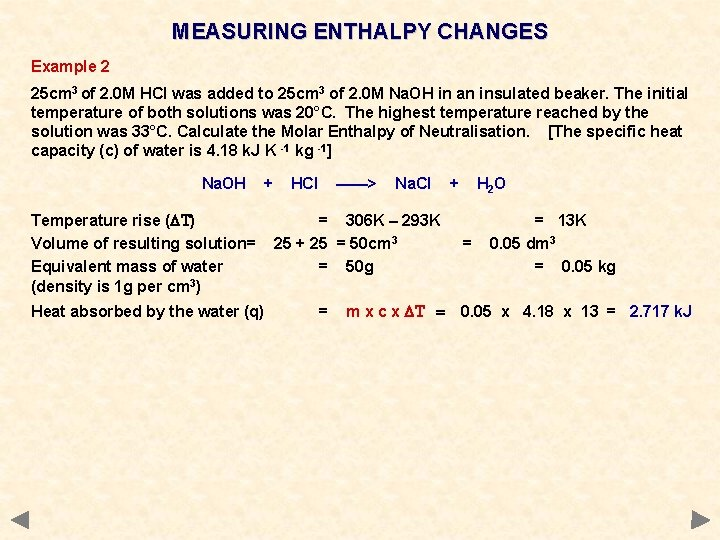MEASURING ENTHALPY CHANGES Example 2 25 cm 3 of 2. 0 M HCl was added to 25 cm 3 of 2. 0 M Na. OH in an insulated beaker. The initial temperature of both solutions was 20°C. The highest temperature reached by the solution was 33°C. Calculate the Molar Enthalpy of Neutralisation. [The specific heat capacity (c) of water is 4. 18 k. J K -1 kg -1] Na. OH + Temperature rise (DT) Volume of resulting solution= Equivalent mass of water (density is 1 g per cm 3) Heat absorbed by the water (q) HCl ——> Na. Cl = 306 K – 293 K 25 + 25 = 50 cm 3 = 50 g = m x c x DT = + H 2 O = = 13 K 0. 05 dm 3 = 0. 05 kg 0. 05 x 4. 18 x 13 = 2. 717 k. J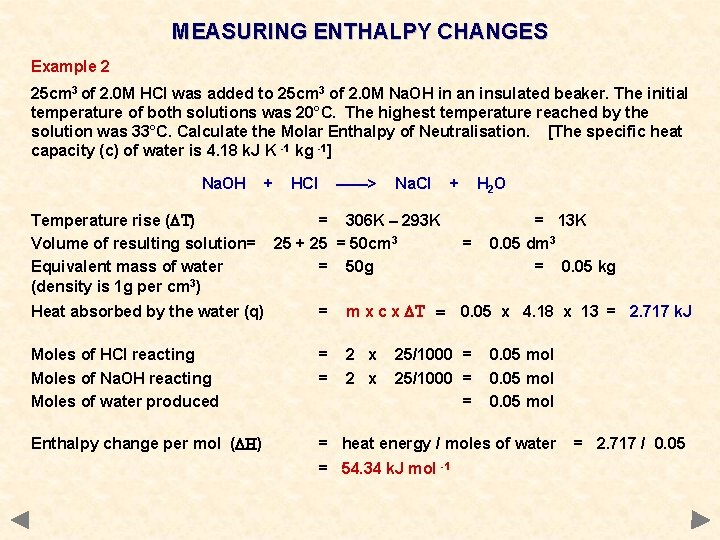MEASURING ENTHALPY CHANGES Example 2 25 cm 3 of 2. 0 M HCl was added to 25 cm 3 of 2. 0 M Na. OH in an insulated beaker. The initial temperature of both solutions was 20°C. The highest temperature reached by the solution was 33°C. Calculate the Molar Enthalpy of Neutralisation. [The specific heat capacity (c) of water is 4. 18 k. J K -1 kg -1] Na. OH + Temperature rise (DT) Volume of resulting solution= Equivalent mass of water (density is 1 g per cm 3) HCl ——> Na. Cl + = 306 K – 293 K 25 + 25 = 50 cm 3 = 50 g H 2 O = = 13 K 0. 05 dm 3 = 0. 05 kg Heat absorbed by the water (q) = m x c x DT = Moles of HCl reacting Moles of Na. OH reacting Moles of water produced = = 2 x Enthalpy change per mol (DH) = heat energy / moles of water 0. 05 x 4. 18 x 13 = 2. 717 k. J 25/1000 = = = 54. 34 k. J mol -1 0. 05 mol = 2. 717 / 0. 05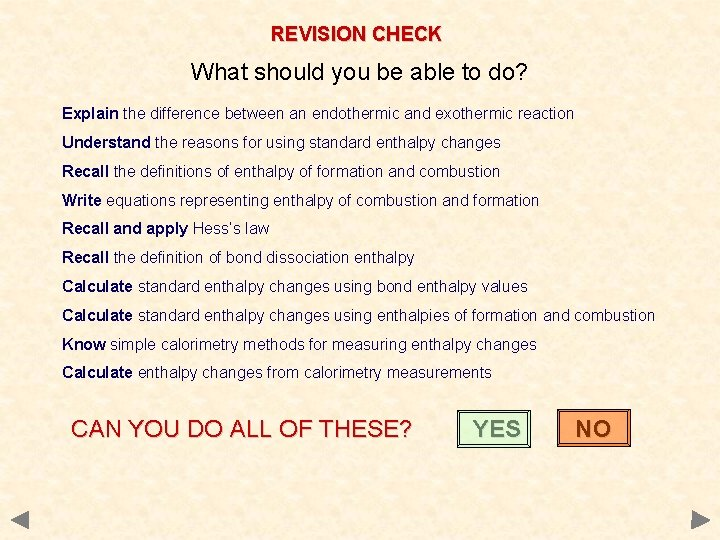REVISION CHECK What should you be able to do? Explain the difference between an endothermic and exothermic reaction Understand the reasons for using standard enthalpy changes Recall the definitions of enthalpy of formation and combustion Write equations representing enthalpy of combustion and formation Recall and apply Hess’s law Recall the definition of bond dissociation enthalpy Calculate standard enthalpy changes using bond enthalpy values Calculate standard enthalpy changes using enthalpies of formation and combustion Know simple calorimetry methods for measuring enthalpy changes Calculate enthalpy changes from calorimetry measurements CAN YOU DO ALL OF THESE? YES NOYou need to go over the relevant topic(s) again Click on the button to return to the menuWELL DONE! Try some past paper questionsENTHALPY CHANGES THE END © 2003 JONATHAN HOPTON & KNOCKHARDY PUBLISHING